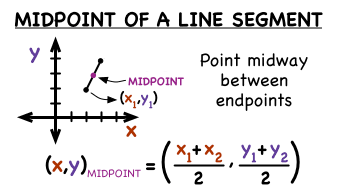How do you find the midpoint between (-3,-1), (-11,3)?

May 28, 2018

$\left(- 7 , 1\right)$

Explanation:

The midpoint formula is shown here:We are given the two endpoints, so we can plug it into the formula to find the midpoint. Notice that the formula is same as the average of the two x-values and y-values.

$\text{Midpoint} = \left(\frac{- 3 + \left(- 11\right)}{2} , \frac{- 1 + 3}{2}\right)$

$\quad \quad \quad \quad \quad \quad \quad \quad = \left(\frac{- 14}{2} , \frac{2}{2}\right)$

$\quad \quad \quad \quad \quad \quad \quad \quad = \left(- 7 , 1\right)$

Hope this helps!